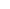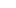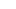Public Health – Statistical Analysis - Research Prospect# Public Health – Statistical Analysis

Here is a sample that showcases why we are one of the world’s leading academic writing firms. This assignment was created by one of our expert academic writers and demonstrated the highest academic quality. Place your order today to achieve academic greatness.

## Introduction

This is a statistical report carried out to analyse the impact of polio and whooping cough vaccination programs over the macroeconomic indicators in Columbia and Mexico from 1950 to 1990. This objective is tested out to improve the macroeconomic indicators specifically for Mexico and Columbia in terms of morbidity and mortality.

## Methodology

To fulfil the objective of the research, there are different statistical tests have been applied. To run the tests efficiently, the software of STATA has been used. Three tests are specifically used: ARIMA, logistic regression model, and sensitivity analysis. To analyse the data, it is essential to differentiate among the research’s independent and dependent variables. For the data set under consideration for this research, the dependent variables are PIB per capita, human development index, and life expectancy. On the other hand, the independent variables of the research are morbidity, mortality, demographics, vaccination coverage, and whooping cough.

## Data Analysis

### ARIMA

ARIMA is an essential acronym for Autoregressive Integrated Moving Average, used to analyse the time-series data to predict future points (Baum, 2004). Firstly, ARIMA has been carried out for Mexico and Columbia to analyse the above independent and dependent variables concerning countries and diseases (Polio and Whooping Cough).The above table shows ARIMA, which is carried out for Mexico. This model determines whether each term is significant or not, which can be predicted by the sig value, which should be lower than 0.05. Based on the considerable value, it can be said that only demographics is significant in the model with respect to PIB per capita because its probability value is 0.033.The above table shows ARIMA, which is carried out for Mexico with respect to life expectancy. Based on the significant value, it can be said that morbidity and mortality are significant in the model with respect to life expectancy because their probability values are 0.001 and 0.000, respectively.The above table shows ARIMA, which is carried out for Mexico with respect to Human Development Index. Based on the significant value, polio and demographics are substantial in the model with respect to the human development index because their probability values are 0.000 and 0.000, respectively.

ARIMA have been carried out for Mexico with respect to Whooping cough as well, the following section include the results by taking whooping cough as one of the independent variables:The above table shows ARIMA, which is carried out for Mexico with respect to PIB per capita. This model determines whether each term is significant or not, which can be predicted by the sig value, which should be lower than 0.05. Based on the considerable value, it can be said that morbidity and whooping cough are significant in the model with respect to PIB per capita because their probability values are 0.000 and 0.003, respectively.The above table shows ARIMA, which is carried out for Mexico with respect to life expectancy. Based on the significant value, mortality and demographics are substantial in the model with respect to life expectancy because their probability values are 0.000 and 0.000, respectively.The above table shows ARIMA, which is carried out for Mexico with respect to the human development index. Based on the significant value, it can be said that mortality, morbidity, and demographics are substantial in the model with respect to the human development index because their probability values are 0.000, 0.000, and 0.000, respectively.

The following section shows the analysis of ARIMA, which has been carried out for country two, Columbia, for the two diseases that are into consideration: polio and whooping cough.The above table shows ARIMA, which is carried out for Columbia with respect to PIB per capita for the disease polio. Based on the significant value, only demographics are substantial in the model with respect to PIB per capita because its probability value is 0.000.The above table shows ARIMA, which is carried out for Columbia with respect to life expectancy for the disease polio. Based on the significant value, only demographics are substantial in the model with respect to life expectancy because their probability value is 0.000.The above table shows ARIMA, which is carried out for Columbia with respect to Human Development Index for polio. Based on the significant value, mortality, polio, and demographics are substantial in the model with respect to the human development index because their probability values are 0.000, 0.000 and 0.000, respectively.The above table shows ARIMA, which is carried out for Columbia with respect to PIB per capita for the whooping disease cough. This model determines whether each term is significant or not, which can be predicted by the sig value, which should be lower than 0.05. Based on the significant value, it can be said that only demographics is substantial in the model with respect to PIB per capita because its probability value is 0.000.The above table shows ARIMA, which is carried out for Columbia with respect to life expectancy for the disease whooping. This model determines whether each term is significant or not, which can be predicted by the sig value, which should be lower than 0.05. Based on the considerable value, only demographics are substantial in the model with respect to life expectancy because their probability value is 0.000.## Hire an Expert Dissertation Writer

Orders completed by our expert writers are

• Formally drafted in an academic style
• Free Amendments and 100% Plagiarism Free – or your money back!
• 100% Confidential and Timely Delivery!
• Free anti-plagiarism report
• Appreciated by thousands of clients. Check client reviewsThe above table shows ARIMA, which is carried out for Columbia with respect to Human Development Index for whooping cough. This model determines whether each term is significant or not, which can be predicted by the sig value, which should be lower than 0.05. Based on the considerable value, it can be said that mortality, polio, and demographics are significant in the model with respect to the human development index because their probability values are 0.000, 0.000, and 0.000, respectively.

## Regression

Regression is carried out to determine the impact of the independent variables on the dependent variables of the research (Hamilton, 2012). The following section includes the regression analysis carried out separately for Mexico and Columbia for polio and whooping cough.In the above table, the regression analysis results are shown for Mexico to determine the impact of PIB per capita on morbidity, mortality, polio, and demographics. To signify the relationship among the research’s independent and dependent variables, the p-value should be less than 0.05. The value of R-square determines that the independent variables can determine 94.03% of the changes caused in PIB per capita. The sig value for the model is also less than 0.05; hence there is a significant relationship among independent variables and PIB per capita for Mexico.In the above table, the regression analysis results are shown for Mexico to determine the impact of life expectancy on morbidity, mortality, polio, and demographics. The value of R-square determines that the independent variables can determine 98.34% of the changes caused in life expectancy. The sig value for the model is also less than 0.05; hence there is a significant relationship among independent variables and life expectancy for Mexico.In the above table, the regression analysis results are shown for Mexico to determine the impact of human development on morbidity, mortality, polio, and demographics. The value of R-square determines that the independent variables can determine 98.84% of the changes caused in the human development index. The sig value for the model is also less than 0.05; hence there is a significant relationship among independent variables and the human development index for Mexico.In the above table, the regression analysis results are shown for Mexico to determine the impact of PIB per capita on morbidity, mortality, whooping cough, and demographics. To signify the relationship among the research’s independent and dependent variables, the p-value should be less than 0.05. The value of R-square determines that the independent variables can determine 91.37% of the changes caused in PIB per capita. The sig value for the model is also less than 0.05; hence there is a significant relationship among independent variables and PIB per capita for Mexico.In the above table, the regression analysis results are shown for Mexico to determine the impact of life expectancy on morbidity, mortality, whooping, and demographics. The value of R-square determines that the independent variables can determine 99.59% of the changes caused in life expectancy. The sig value for the model is also less than 0.05; hence there is a significant relationship among independent variables and life expectancy for Mexico.In the above table, the regression analysis results are shown for Mexico to determine the impact of human development on morbidity, mortality, whooping cough, and demographics. The value of R-square determines that the independent variables can determine 99.39% of the changes caused in the human development index. The sig value for the model is also less than 0.05; hence there is a significant relationship among independent variables and the human development index for Mexico.

The following section shows the results of regression for the second country that is Columbia, for the diseases taken into consideration: polio and whooping cough.In the above table, the regression analysis results are shown for Columbia to determine the impact of PIB per capita on morbidity, mortality, polio, and demographics. The value of R-square determines that the independent variables can determine 98.54% of the changes caused in PIB per capita. The sig value for the model is also less than 0.05; hence there is a significant relationship among independent variables and PIB per capita for Columbia.In the above table, the regression analysis results are shown for Columbia to determine the impact of life expectancy on morbidity, mortality, polio, and demographics. The value of R-square determines that the independent variables can determine 95.37% of the changes caused in life expectancy. The sig value for the model is also less than 0.05; hence there is a significant relationship among independent variables and life expectancy for Columbia.

In the above table, the regression analysis results are shown for Columbia to determine the impact of human development on morbidity, mortality, polio, and demographics. The value of R-square determines that the independent variables can determine 99.71% of the changes caused in the human development index. The sig value for the model is also less than 0.05; hence there is a significant relationship among independent variables and the human development index for Columbia.

In the above table, the regression analysis results are shown for Columbia to determine the impact of PIB per capita on morbidity, mortality, whooping cough, and demographics. The value of R-square determines that the independent variables can determine 98.46% of the changes caused in PIB per capita. The sig value for the model is also less than 0.05; hence there is a significant relationship among independent variables and PIB per capita for Columbia.

In the above table, the regression analysis results are shown for Columbia to determine the impact of life expectancy on morbidity, mortality, whooping, and demographics. The value of R-square determines that the independent variables can determine 95.34% of the changes caused in life expectancy. The sig value for the model is also less than 0.05; hence there is a significant relationship among independent variables and life expectancy for Columbia.In the above table, the regression analysis results are shown for Columbia to determine the impact of human development on morbidity, mortality, whooping cough, and demographics. The value of R-square determines that the independent variables can determine 99.72% of the changes caused in the human development index. The sig value for the model is also less than 0.05; hence there is a significant relationship among independent variables and the human development index for Columbia.In the above table, the regression analysis results are shown for Columbia to determine the impact of PIB per capita on morbidity, mortality, whooping cough, and demographics. The value of R-square determines that the independent variables can determine 98.46% of the changes caused in PIB per capita. The sig value for the model is also less than 0.05; hence there is a significant relationship among independent variables and PIB per capita for Columbia.In the above table, the regression analysis results are shown for Columbia to determine the impact of life expectancy on morbidity, mortality, whooping, and demographics. The value of R-square determines that the independent variables can determine 95.34% of the changes caused in life expectancy. The sig value for the model is also less than 0.05; hence there is a significant relationship among independent variables and life expectancy for Columbia.In the above table, the regression analysis results are shown for Columbia to determine the impact of human development on morbidity, mortality, whooping cough, and demographics. The value of R-square determines that the independent variables can determine 99.72% of the changes caused in the human development index. The sig value for the model is also less than 0.05; hence there is a significant relationship among independent variables and the human development index for Columbia.

## Sensitivity Analysis

It is carried out to assess the impact of values of independent variables on the values of a dependent variable. Sensitivity analysis for this data set is carried out to assess polio and whooping cough vaccination programs on the macroeconomic indicators in Columbia and Mexico. Following are the results:## Conclusion

To sum up, the selected statistical tests have yielded significant results which show the impact of independent variables on dependent variables of the research. Moreover, it has been identified with the help of the statistical results that different vaccination programs for polio and whooping cough in Mexico and Columbia can help improve macroeconomic indicators.

## References

Baum, C.F., 2004. Stata: The language of choice for time series analysis?. Working Papers in Economics, p.4.

Hamilton, L.C., 2012. Statistics with Stata: version 12. Cengage Learning.